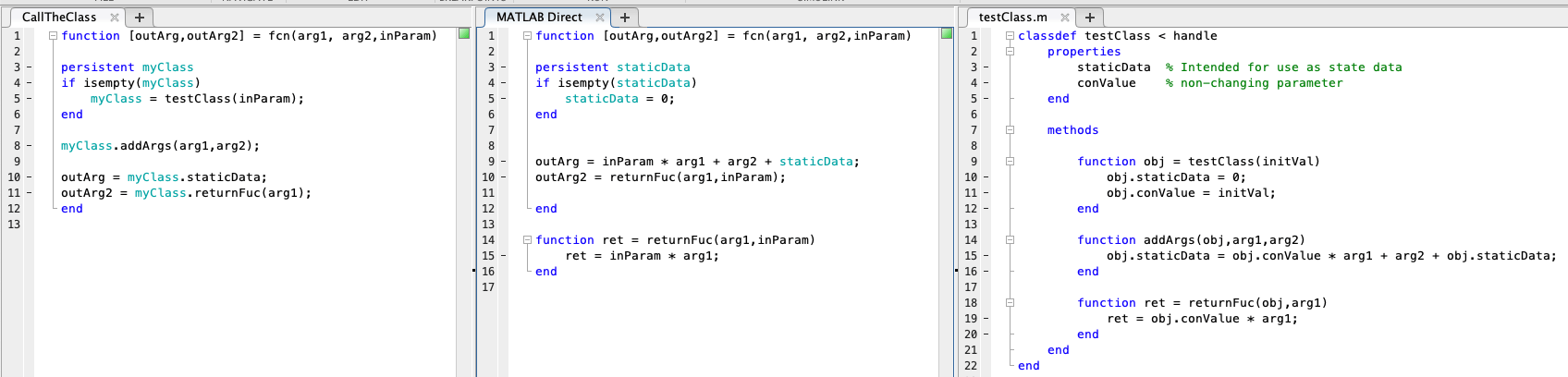## Simulink has Class

Simulink is a graphical control design environment. However, it has the ability to include MATLAB, textual-based, algorithms as part of the model. For some design problems, this is the best approach. In today’s post, I’m going to review the three primary methods for including MATLAB code in your Simulink Model.

## Function / Class / System Object

There are three methods for including MATLAB code into Simulink Models; a function, MATLAB classes, or MATLAB Simulink System Objects.

The Class and System Objects provide functionality that is not built into the Function method. However, both require additional knowledge of how to program. A MATLAB function can be written as a simple set of equations while the Object Oriented methods require some base level programing knowlege.

## And state data: memory

For models that are targeting Code Generation there is an additional consideration, memory usage. State data, or dWork data in the generated code is used for any variable that is required to be held in memory between time steps. With a MATLAB function the user can explicitly define the variables that are State Data by using the “persistent” keyword.Example of calling a MATLAB class from within a MATLAB function block in Simulink

With the MATLAB class or MATLAB System Object, any data that is defined as a property will be stored as a dWork variable regardless of the need for a state variable. The number of state variables can be decreased in the Class implementation; however in doing so, much of the benefit of the class based approach is lost.

This site uses Akismet to reduce spam. Learn how your comment data is processed.